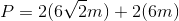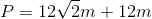# High School Math : How to find the perimeter of kite

## Example Questions

### Example Question #1 : How To Find The Perimeter Of Kite

Find the perimeter of the following kite: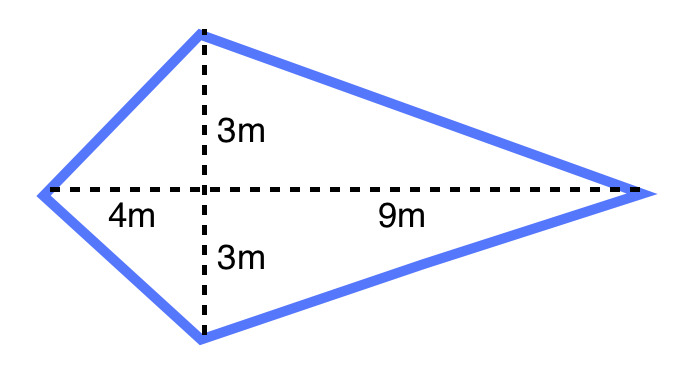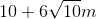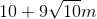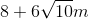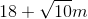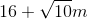Explanation:

In order to find the length of the two shorter edges, use a Pythagorean triple: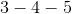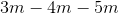In order to find the length of the two longer edges, use the Pythagorean theorem: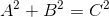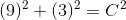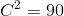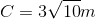The formula of the perimeter of a kite is: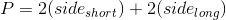Plugging in our values, we get: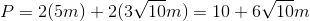### Example Question #2 : How To Find The Perimeter Of Kite

Find the perimeter of the following kite: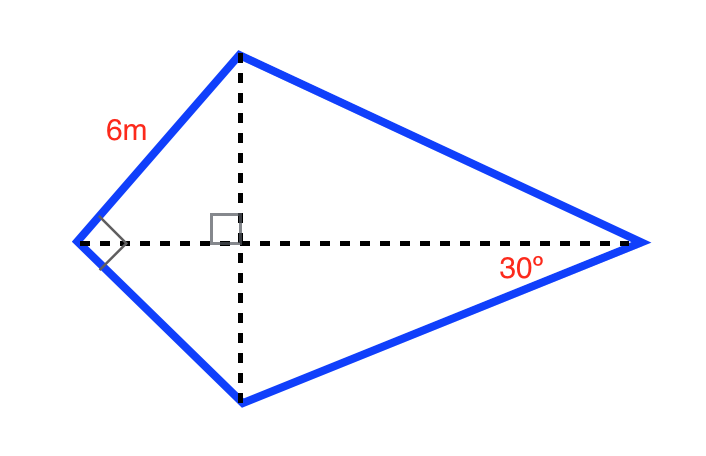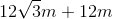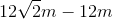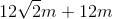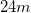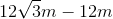Explanation:

The formula for the perimeter of a kite is: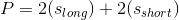Where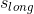is the length of the longer side and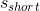is the length of the shorter side

Use the formulas for a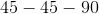triangle and a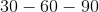triangle to find the lengths of the longer sides. The formula for atriangle is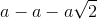and the formula for atriangle is.

Ourtriangle is: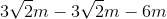Ourtriangle is: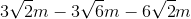Plugging in our values, we get: